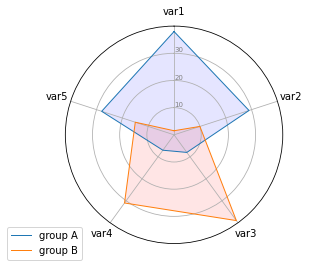This example follows the chart #390 where only one individual was plotted on the radar chart. Once you understood the method, it is quite easy to apply it to more individuals.
Note that if you have more than 2 or 3 individuals, I strongly advise to use faceting instead of displaying all of them on the same plot: spider charts become quickly unreadable.

``````# Libraries
import matplotlib.pyplot as plt
import pandas as pd
from math import pi

# Set data
df = pd.DataFrame({
'group': ['A','B','C','D'],
'var1': [38, 1.5, 30, 4],
'var2': [29, 10, 9, 34],
'var3': [8, 39, 23, 24],
'var4': [7, 31, 33, 14],
'var5': [28, 15, 32, 14]
})

# ------- PART 1: Create background

# number of variable
categories=list(df)[1:]
N = len(categories)

# What will be the angle of each axis in the plot? (we divide the plot / number of variable)
angles = [n / float(N) * 2 * pi for n in range(N)]
angles += angles[:1]

# Initialise the spider plot
ax = plt.subplot(111, polar=True)

# If you want the first axis to be on top:
ax.set_theta_offset(pi / 2)
ax.set_theta_direction(-1)

# Draw one axe per variable + add labels
plt.xticks(angles[:-1], categories)

# Draw ylabels
ax.set_rlabel_position(0)
plt.yticks([10,20,30], ["10","20","30"], color="grey", size=7)
plt.ylim(0,40)

# ------- PART 2: Add plots

# Plot each individual = each line of the data
# I don't make a loop, because plotting more than 3 groups makes the chart unreadable

# Ind1
values=df.loc.drop('group').values.flatten().tolist()
values += values[:1]
ax.plot(angles, values, linewidth=1, linestyle='solid', label="group A")
ax.fill(angles, values, 'b', alpha=0.1)

# Ind2
values=df.loc.drop('group').values.flatten().tolist()
values += values[:1]
ax.plot(angles, values, linewidth=1, linestyle='solid', label="group B")
ax.fill(angles, values, 'r', alpha=0.1)